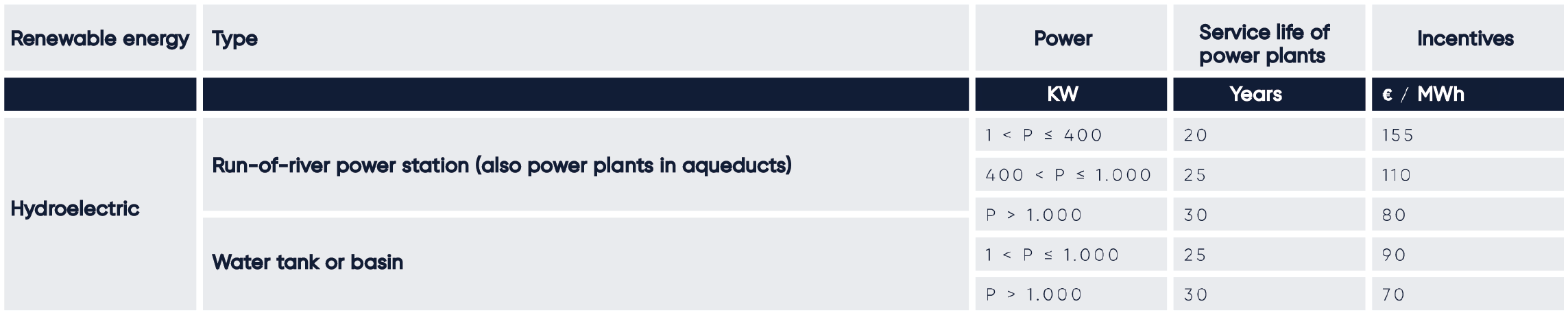## HYDROELECTRIC CALCULATION

### ONLINE HYDROELECTRIC CALCULATION

The power of Hydroelectric plant depends on:

• – Water height difference between water resource height and water level at the end of the system;
• – Water flow rate that is the mass of water in a unit time.

Different powers:

• micro plants P<100 kWp
• mini plants 100 kWp < P < 1 MWp
• small plants 1 MWp < P < 10 MWp
• large plants P > 10 MWp.

Different heights:

• small height H < 20 m
• medium height 20 m < H < 100 m
• large height 100 m < H < 1000 m
• very large height H > 1000 m.

Different flow rates:

• small flow rate Q < 10 m3/s
• medium flow rate 10 m3/s < Q < 100 m3/s
• large flow rate 100 m3/s < Q < 1000 m3/s
• very large flow rate Q > 1000 m3/s.• m
• m3/s
• kW
• %
• kW
• Max 8,700 h
• kWh
• €/kWh